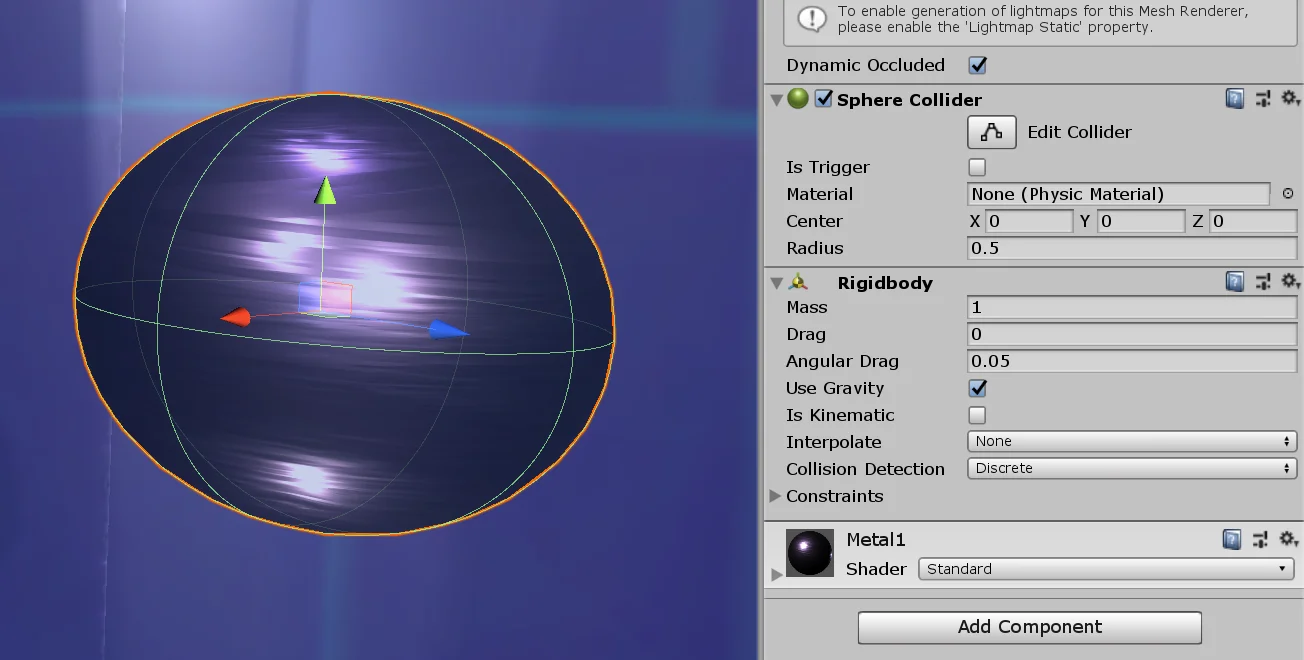# #7 AddForce Method in Unity. RigidBody Class

## Introduction

In this article we are going to study how to use the AddForce method of Unity’s RigidBody class, which allows us to apply forces to GameObjects that have a RigidBody component assigned to them. The goal is to make the ball in the GameDevLab bounce indefinitely for the duration of the game.

## Procedure

We are going to use the “AddForce” station, which consists of a diamond-shaped container with a ball inside. The objective is to make the ball bounce indefinitely inside the station. To do this we must apply a force when the ball hits the bottom.

In the hierarchy we have the GameObject “#7 AddForce” that contains all the elements of the station (figure 2). This GameObject is also assigned the Script “AddForceToObject”.

In figure 3 we see the “AddForceToObject” component in the inspector. We can assign an object to which to apply the force, in this case is “Sphere” the ball that is inside the station. We can assign a minimum and maximum force value to apply to the ball and the direction of the force.

With the purpose of learning a little about what a force is and how it is defined I made this video (activate english subtitles):Fig. 4: To the left, the sphere of the “AddForce” station. On the right, its components are displayed in the inspector. Between them there is a RigidBody component that will give you physical behavior.

The Ball GameObject is simply a primitive sphere made in Unity, it is assigned a RigidBody component that gives it physical behavior. The ball will be affected by gravity, it will be able to collide with objects that Colliders have assigned and we will be able to apply forces to it.

At the base of the structure we have a cylinder-shaped GameObject that has a Box Collider assigned in Trigger mode (figure 4). It also has a script called “AddForceCollisionDetection” that will detect the ball.

## Solution

When we open the “AddForceToObject” Script for the first time we find what you see in figure 6. All the parameters you see in the inspector are defined and it contains only one method called “addForce”. This method is defined as public because it is the one that is going to be called when it is detected that the ball has touched the ground of the structure.

The solution of this exercise is shown below and the purpose of each instruction is explained.

To solve this problem we will require three instructions. The first will define the magnitude of the force to be applied, which will be a random value between the minimum and maximum forces.

The second instruction will define the total force to apply, if you saw the video about physics, to define a force we need a magnitude and a direction. For the direction we use the vector of three dimensions (class Vector3) that is seen in the inspector, it is called “forceDirectionVector”. But we are not going to use it as it is written in the inspector. To prevent that Vector3 from affecting the magnitude of the force we must normalize it. We do this with the “Normalize” method of the Vector3 class. The total force to apply will be a Vector3 called “force” which is the result of multiplying the direction by the magnitude.

Finally the third instruction consists in applying force on the object. The forces are applicable to GameObjects that have a RigidBody component assigned, in our case we are going to apply the force on the RigidBody component of the ball. We use the “GetComponent” method to obtain the RigidBody component and finally we execute the AddForce method, passing as parameter the force to apply.